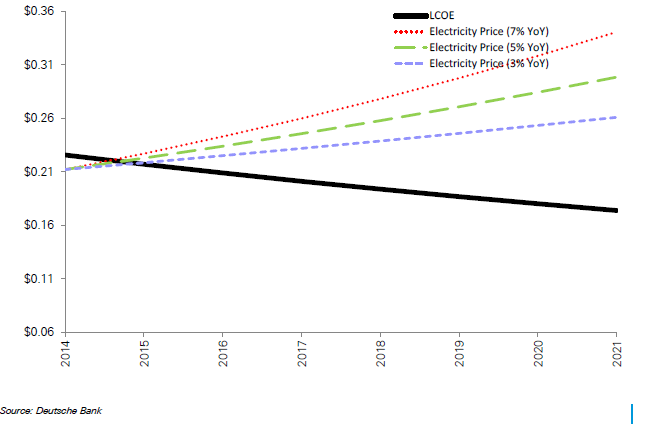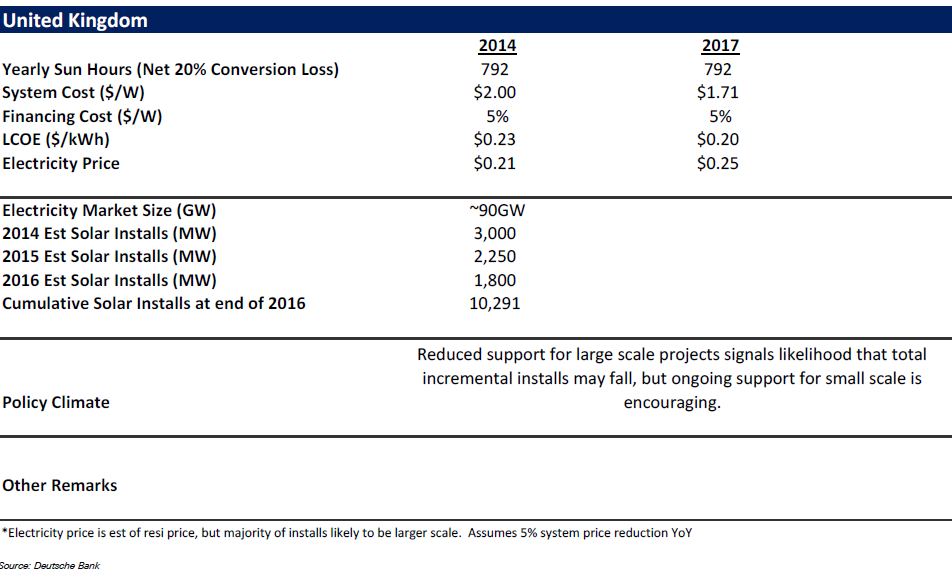The cost of power generated from rooftop solar panels has been the subject of intense debate worldwide and in the UK too.

With cost of solar power fast decreasing, millions of residential and commercial units in the UK are interested to know the actual cost of rooftop solar power in the UK.

There are two costs, when we talk of solar power. One is the unsubsidized cost, and the other is the cost of solar power after subsidies.

Usually, the cost of solar power is denoted as LCOE, which stands for levelized cost of electricity. This metric, while it sounds complex, is a fairly simple one:

LCOE = total costs during the entire project cycle/total units of electricity generated during the entire project lifecycle.

Solar power systems have a lifespan of 25 years. Thus, while calculating LCOE, we need to estimate all the costs (capital costs, financing costs, O&M costs, costs for parts replacement etc.) and estimate the total number of units of electricity that will be produced during the 25 years of the project, and divide the former by the latter.

The LCOE gives the true cost for a solar power system which has high upfront costs but provides benefits over 25 years.

 Based on analyses from a number of sources, we estimate that the levelized cost of solar power in the UK in 2015 is in the range 20-23 US cents/kWh ( 13-15 p/kWh). What is the projected reduction in the cost of rooftop solar electricity? Although forecasts have generally been accurate about the downward trend of PV costs, there still lies some considerable uncertainty about the exact level of cost reduction the sector will experience or by when, and even small changes can affect long-term estimates of cost reductions. Going by an analysis of estimates, Solar Mango predicts that there will be further reduction of 25% between 2015 and 2020. Note that here we consider rooftop solar to be in the <4 KW category.

Sources & References for Cost of Solar Power in the UK

UK LCOE Scenario AnalysisSource: : https://www.db.com/cr/en/docs/solar_report_full_length.pdf

Subscribe to FREE Solar Mango Newsletter - News and Opinions on Implementing Solar

Related Articles